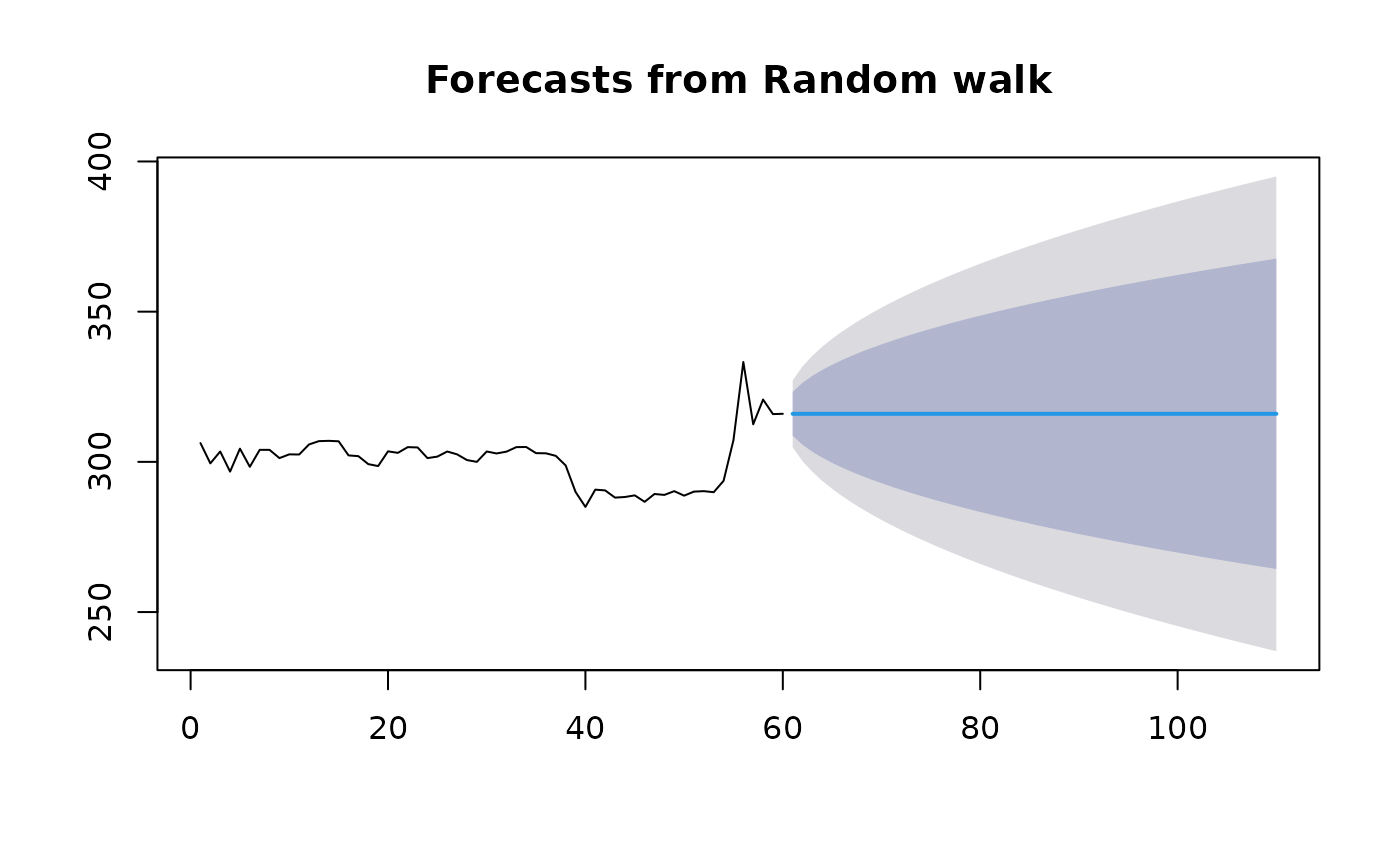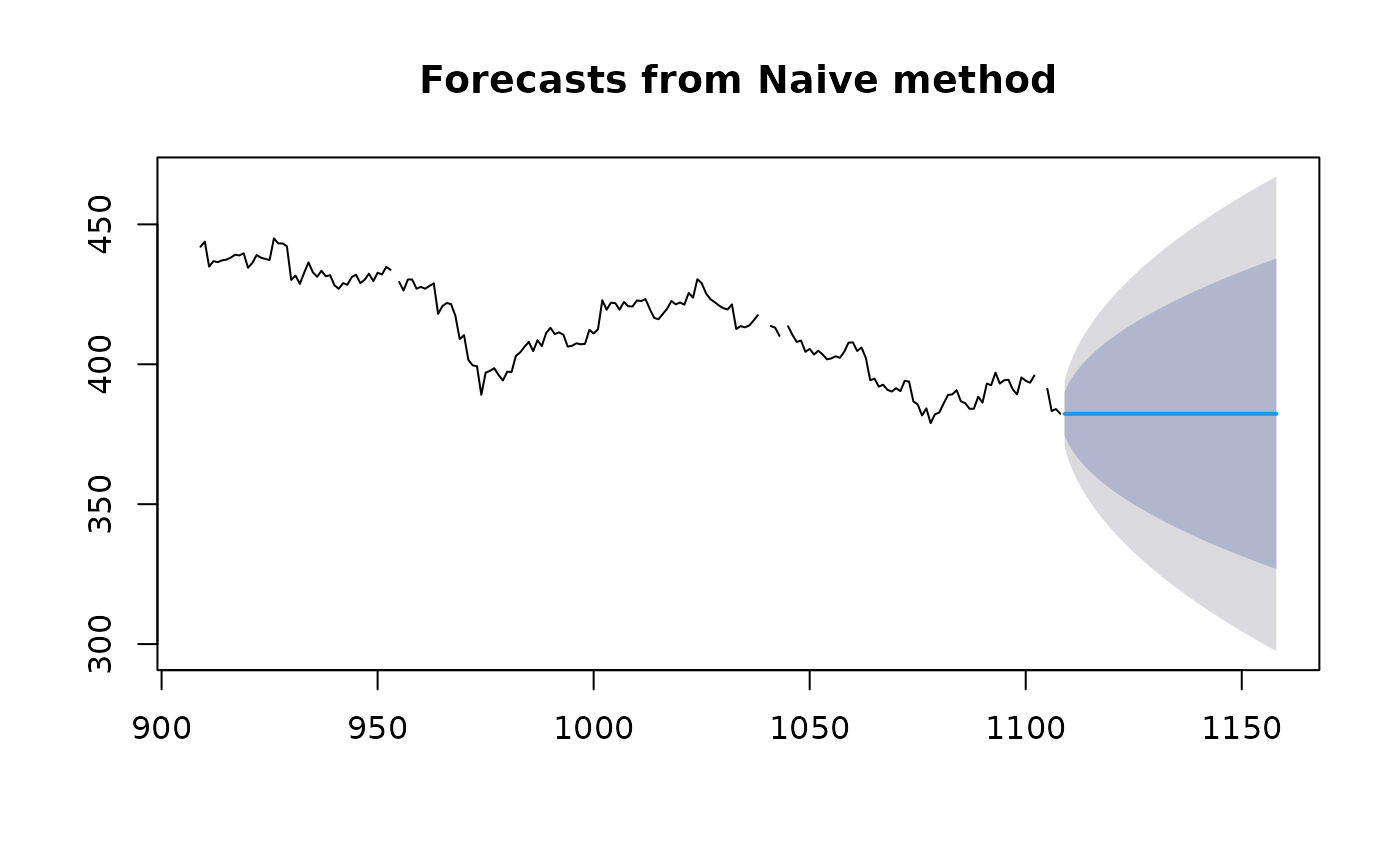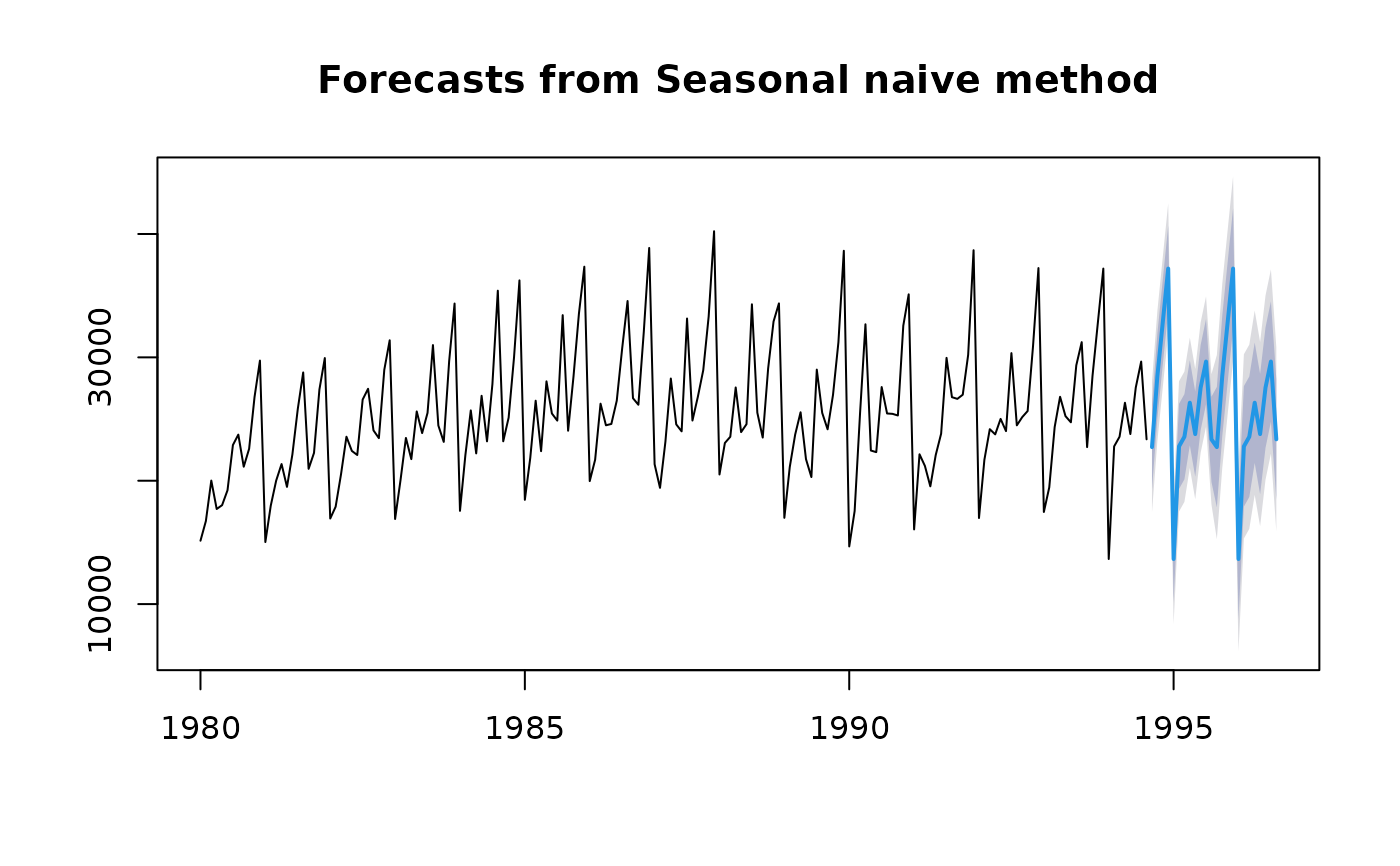rwf() returns forecasts and prediction intervals for a random walk with drift model applied to y. This is equivalent to an ARIMA(0,1,0) model with an optional drift coefficient. naive() is simply a wrapper to rwf() for simplicity. snaive() returns forecasts and prediction intervals from an ARIMA(0,0,0)(0,1,0)m model where m is the seasonal period.

## Usage

rwf(
y,
h = 10,
drift = FALSE,
level = c(80, 95),
fan = FALSE,
lambda = NULL,
...,
x = y
)

naive(
y,
h = 10,
level = c(80, 95),
fan = FALSE,
lambda = NULL,
...,
x = y
)

snaive(
y,
h = 2 * frequency(x),
level = c(80, 95),
fan = FALSE,
lambda = NULL,
...,
x = y
)

## Arguments

y

a numeric vector or time series of class ts

h

Number of periods for forecasting

drift

Logical flag. If TRUE, fits a random walk with drift model.

level

Confidence levels for prediction intervals.

fan

If TRUE, level is set to seq(51,99,by=3). This is suitable for fan plots.

lambda

Box-Cox transformation parameter. If lambda="auto", then a transformation is automatically selected using BoxCox.lambda. The transformation is ignored if NULL. Otherwise, data transformed before model is estimated.

Use adjusted back-transformed mean for Box-Cox transformations. If transformed data is used to produce forecasts and fitted values, a regular back transformation will result in median forecasts. If biasadj is TRUE, an adjustment will be made to produce mean forecasts and fitted values.

...

Additional arguments affecting the forecasts produced. If model=NULL, forecast.ts passes these to ets or stlf depending on the frequency of the time series. If model is not NULL, the arguments are passed to the relevant modelling function.

x

Deprecated. Included for backwards compatibility.

## Value

An object of class "forecast".

The function summary is used to obtain and print a summary of the results, while the function plot produces a plot of the forecasts and prediction intervals.

The generic accessor functions fitted.values and residuals

extract useful features of the value returned by naive or snaive.

An object of class "forecast" is a list containing at least the following elements:

model

A list containing information about the fitted model

method

The name of the forecasting method as a character string

mean

Point forecasts as a time series

lower

Lower limits for prediction intervals

upper

Upper limits for prediction intervals

level

The confidence values associated with the prediction intervals

x

The original time series (either object itself or the time series used to create the model stored as object).

residuals

Residuals from the fitted model. That is x minus fitted values.

fitted

Fitted values (one-step forecasts)

## Details

The random walk with drift model is $$Y_t=c + Y_{t-1} + Z_t$$ where $$Z_t$$ is a normal iid error. Forecasts are given by $$Y_n(h)=ch+Y_n$$. If there is no drift (as in naive), the drift parameter c=0. Forecast standard errors allow for uncertainty in estimating the drift parameter (unlike the corresponding forecasts obtained by fitting an ARIMA model directly).

The seasonal naive model is $$Y_t= Y_{t-m} + Z_t$$ where $$Z_t$$ is a normal iid error.

Arima

Rob J Hyndman

## Examples


gold.fcast <- rwf(gold[1:60], h=50)
plot(gold.fcast)plot(naive(gold,h=50),include=200)plot(snaive(wineind))